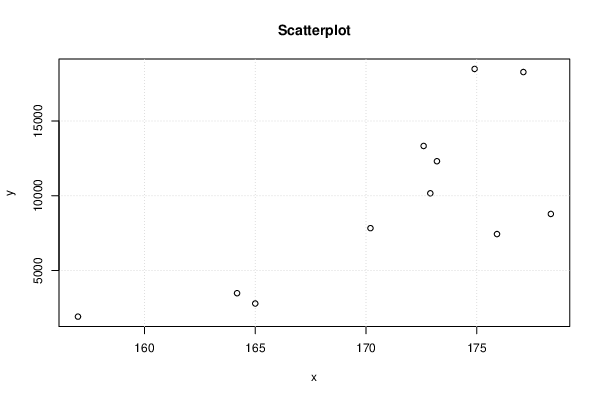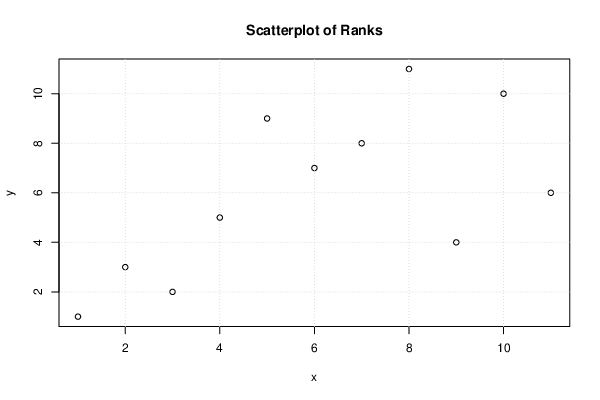## Free Statistics

of Irreproducible Research!

Author's title
Author*Unverified author*
R Software Modulerwasp_spearman.wasp
Title produced by softwareSpearman Rank Correlation
Date of computationFri, 07 Feb 2020 13:07:23 +0100
Cite this page as followsStatistical Computations at FreeStatistics.org, Office for Research Development and Education, URL https://freestatistics.org/blog/index.php?v=date/2020/Feb/07/t1581077811jmovuj87po7id6n.htm/, Retrieved Wed, 21 Apr 2021 09:05:07 +0000
Statistical Computations at FreeStatistics.org, Office for Research Development and Education, URL https://freestatistics.org/blog/index.php?pk=319069, Retrieved Wed, 21 Apr 2021 09:05:07 +0000
QR Codes:Original text written by user:
IsPrivate?No (this computation is public)
User-defined keywords
Estimated Impact44
Family? (F = Feedback message, R = changed R code, M = changed R Module, P = changed Parameters, D = changed Data)
-       [Spearman Rank Correlation] [] [2020-02-07 12:07:23] [d41d8cd98f00b204e9800998ecf8427e] [Current]
Feedback Forum

Post a new message
Dataseries X:
157
165
164.18
170.2
172.9
173.2
172.6
174.9
175.91
178.34
177.10
Dataseries Y:
1922
2800
3489.5
7835
10165
12309
13327,17
18474
7440,4
8785
18267

 Summary of computational transaction Raw Input view raw input (R code) Raw Output view raw output of R engine Computing time 0 seconds R Server Big Analytics Cloud Computing Center

\begin{tabular}{lllllllll}
\hline
Summary of computational transaction \tabularnewline
Raw Input view raw input (R code)  \tabularnewline
Raw Outputview raw output of R engine  \tabularnewline
Computing time0 seconds \tabularnewline
R ServerBig Analytics Cloud Computing Center \tabularnewline
\hline
\end{tabular}
%Source: https://freestatistics.org/blog/index.php?pk=319069&T=0

[TABLE]
[ROW]
 Summary of computational transaction[/C][/ROW] [ROW] Raw Input[/C] view raw input (R code) [/C][/ROW] [ROW] Raw Output[/C] view raw output of R engine [/C][/ROW] [ROW] Computing time[/C] 0 seconds[/C][/ROW] [ROW] R Server[/C] Big Analytics Cloud Computing Center[/C][/ROW] [/TABLE] Source: https://freestatistics.org/blog/index.php?pk=319069&T=0

Globally Unique Identifier (entire table): ba.freestatistics.org/blog/index.php?pk=319069&T=0

As an alternative you can also use a QR Code:

The GUIDs for individual cells are displayed in the table below:

 Summary of computational transaction Raw Input view raw input (R code) Raw Output view raw output of R engine Computing time 0 seconds R Server Big Analytics Cloud Computing Center

 Spearman Rank Correlation rho 0.636363636363636 2-sided p-value 0.0403864039827663 S 80

\begin{tabular}{lllllllll}
\hline
Spearman Rank Correlation \tabularnewline
rho & 0.636363636363636 \tabularnewline
2-sided p-value & 0.0403864039827663 \tabularnewline
S & 80 \tabularnewline
\hline
\end{tabular}
%Source: https://freestatistics.org/blog/index.php?pk=319069&T=1

[TABLE]
[ROW][C]Spearman Rank Correlation[/C][/ROW]
[ROW][C]rho[/C][C]0.636363636363636[/C][/ROW]
[ROW][C]2-sided p-value[/C][C]0.0403864039827663[/C][/ROW]
[ROW][C]S[/C][C]80[/C][/ROW]
[/TABLE]
Source: https://freestatistics.org/blog/index.php?pk=319069&T=1

Globally Unique Identifier (entire table): ba.freestatistics.org/blog/index.php?pk=319069&T=1

As an alternative you can also use a QR Code:

The GUIDs for individual cells are displayed in the table below:

 Spearman Rank Correlation rho 0.636363636363636 2-sided p-value 0.0403864039827663 S 80PNG link Postscript link PDF linkPNG link Postscript link PDF link

Parameters (Session):
Parameters (R input):
R code (references can be found in the software module):
k <- cor.test(x,y,method='spearman')bitmap(file='test1.png')plot(x,y,main='Scatterplot',xlab=xlab,ylab=ylab)grid()dev.off()bitmap(file='test2.png')plot(rank(x),rank(y),main='Scatterplot of Ranks',xlab=xlab,ylab=ylab)grid()dev.off()load(file='createtable')a<-table.start()a<-table.row.start(a)a<-table.element(a,'Spearman Rank Correlation',2,TRUE)a<-table.row.end(a)a<-table.row.start(a)a<-table.element(a,'rho',header=TRUE)a<-table.element(a,k$estimate)a<-table.row.end(a)a<-table.row.start(a)a<-table.element(a,'2-sided p-value',header=TRUE)a<-table.element(a,k$p.value)a<-table.row.end(a)a<-table.row.start(a)a<-table.element(a,'S',header=TRUE)a<-table.element(a,k\$statistic)a<-table.row.end(a)a<-table.end(a)table.save(a,file='mytable.tab')